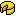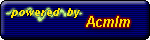Archive
 Register | Login | Logout | Edit profile Views: 1397612 Main | Memberlist | Calendar | Online users Ranks | FAQ | Search | Photo album 11-13-07 11:42 PM
 rustedmagick forums - The ROM Hacking Data Repository - Cutting Room Floor?: Equinox|User
Post
KingMikeBag of Stinky Weeds
Level: 5Posts: 3/5
EXP: 436
For next: 93

Since: 09-12-03

Since last post: 837 days
Last activity: 105 days
 Posted on 02-06-04 03:01 PM Quote
Maybe it had plot stuff later (I played to the third dungeon and found not a line of story). But I cracked open the Japanese ROM (Solstice 2) in a hex editor to find what looked like a whole plotline in Japanese, with English translation included, strangely enough (~ offset 50000-60000 in the ROMs). Both the Japanese and English titles were also in there. The Japanese ROM is also larger by 256KB, yet that extra space seems to be completely empty.
Also, the English appears to have a pre-written ad thing in it (search around offset 5000 in the ROM).

Font table included for reference:
00=0
01=1
02=2
03=3
04=4
05=5
06=6
07=7
08=8
09=9
0A=Ӯ
0B=Ӯў
0C=ӮӨ
0D=ӮҰ
0E=ӮЁ
10=Ӯ«
11=Ӯӯ
12=ӮҜ
13=Ӯұ
14=Ӯі
15=Ӯө
16=Ӯ·
17=Ӯ№
18=Ӯ»
19=ӮҪ
1A=Ӯҝ
1B=ӮВ
1C=ӮД
1D=ӮЖ
1E=ӮИ
1F=ӮЙ
20=ӮК
21=ӮЛ
22=ӮМ
23=ӮН
24=ӮР
25=ӮУ
26=ӮЦ
27=ӮЩ
28=ӮЬ
29=ӮЭ
2A=ӮЮ
2B=ӮЯ
2C=Ӯа
2D=Ӯв
2E=Ӯд
2F=Ӯж
30=Ӯз
31=Ӯи
32=Ӯй
33=Ӯк
34=Ӯл
35=Ӯн
36=Ӯр
37=Ӯс
38=ӮБ
39=Ӯб
3A=Ӯг
3B=Ӯе
3C=Ӯ§
3D=ӮҘ
3E=Ғu
3F=Ғv
40=Ғg
41=Ғh
42=Ғd
43=ҒB
44=ҒA
45=ҒI
46=ғA
47=ғC
48=ғE
49=ғG
4A=ғI
4B=ғJ
4C=ғL
4D=ғN
4E=ғP
4F=ғR
50=ғT
51=ғV
52=ғX
53=ғZ
54=ғ\
55=ғ^
56=ғ`
57=ғc
58=ғe
59=ғg
5A=ғi
5B=ғj
5C=ғk
5D=ғl
5E=ғm
5F=ғn
60=ғq
61=ғt
62=ғw
63=ғz
64=ғ}
65=ғ~
66=ғҖ
67=ғҒ
68=ғӮ
69=ғ„
6A=ғҶ
6B=ғҲ
6C=ғү
6D=ғҠ
6E=ғӢ
6F=ғҢ
70=ғҚ
71=ғҸ
72=ғ’
73=ғ“
74=ғb
75=ғғ
76=ғ…
77=ғҮ
78=ҒH
79=ғB
7A=Ғ[
7B=Ғ`
7C=ӮӘ
7D=Ӯ°
7E=ӮІ
7F=Ӯ¶
80=Ӯё
81=Ӯј
82=Ӯҫ
83=ӮГ
84=ӮЕ
85=ӮЗ
86=ӮО
87=ӮС
88=ӮФ
89=ӮЧ
8A=ӮЪ
8B=ӮП
8C=ӮХ
8D=ӮЫ
8E=ғ@
8F=ғF
90=ғK
91=ғM
92=ғO
93=ғW
94=ғ_
95=ғf
96=ғh
97=ғo
98=ғr
99=ғu
9A=ғx
9B=ғ{
9C=ғ”
9D=ғp
9E=ғs
9F=ғv
A0=ғy
A1=ғ|
A2=ғH
A3=ғQ
A4=ғS
A5=ғU
A6=ғY
A7=ғ[
A8=ғ]
A9=ғa
AA=Ӯ¬
AB=Ӯ®
AC=Ӯҙ
AE=ӮА
AF=ӮТ
B0=ӮШ
B1=ғD
B2=A
B3=B
B4=C
B5=D
B6=E
B7=F
B8=G
B9=H
BA=I
BB=J
BC=K
BD=L
BE=M
BF=N
C0=O
C1=P
C2=Q
C3=R
C4=S
C5=T
C6=U
C7=V
C8=W
C9=X
CA=Y
CB=Z
CC=.
CD=,
CE=:
CF=(
D0=-
D1=*
D2=)
DC=
ScoutboyKeeper of the CheeseKiller of Threads
AdministratorLevel: 51Posts: 116/758
EXP: 947902
For next: 66036

Since: 03-21-02
Rating: 1 (48 pts)

Since last post: 35 days
Last activity: 4 hours
 Posted on 02-06-04 07:35 PM Quote
 Wrong forum.*moves*"History is bunk"

KingMikeBag of Stinky Weeds
Level: 5Posts: 4/5
EXP: 436
For next: 93

Since: 09-12-03

Since last post: 837 days
Last activity: 105 days
 Posted on 02-06-04 10:41 PM Quote
Kinda thought it should've been here, but wasn't sure. Thanks.
BMF54123Guardian of BlobariaNot Scoutboy
AdministratorLevel: 46Posts: 221/602
EXP: 671678
For next: 40096

Since: 03-17-02
From: Spokane, WA

Since last post: 24 days
Last activity: 0 sec.
 Posted on 02-07-04 12:06 AM Quote
 What forum was it originally in? It seems out-of-place here...I really need to make a dedicated Cutting Room Floor forum. :PScoutboyKeeper of the CheeseKiller of Threads
AdministratorLevel: 51Posts: 117/758
EXP: 947902
For next: 66036

Since: 03-21-02
Rating: 1 (48 pts)

Since last post: 35 days
Last activity: 4 hours
 Posted on 02-07-04 08:48 AM Quote
 It was on the COM Port. Due to the data element of it I just put it here for safe keeping."History is bunk"

 rustedmagick forums - The ROM Hacking Data Repository - Cutting Room Floor?: Equinox|rustedmagick.com

AcmlmBoard v1.8a BMF Hax0r Specialҷ